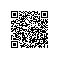# 黑马程序员java笔记之二-----多线程

[java] view plaincopy
2.     private String name ;        // 表示线程的名称
4.         this.name = name ;        // 通过构造方法配置name属性
5.     }
6.     public void run(){    // 覆写run()方法，作为线程 的操作主体
7.         for(int i=0;i<10;i++){
8.             System.out.println(name + "运行，i = " + i) ;
9.         }
10.     }
11.        }
13.         public static void main(String args[]){
16.             mt1.run() ;    // 调用线程主体
17.             mt2.run() ;    // 调用线程主体
18.      }
19. }</span>

[java] view plaincopy
1. class MyThread implements Runnable{    // 实现Runnable接口，作为线程的实现类
2.            private String name ;        // 表示线程的名称
3.
5.             this.name = name ;        // 通过构造方法配置name属性
6.         }
7.         public void run(){    // 覆写run()方法，作为线程 的操作主体
8.             for(int i=0;i<10;i++){
9.                 System.out.println(name + "运行，i = " + i) ;
10.             }
11.         }
12.        }
13.         public class RunnableDemo01{
14.             public static void main(String args[]){
19.                 t1.start() ;    // 启动多线程
20.                 t2.start() ;    // 启动多线程
21.         }
22.     }

[java] view plaincopy
1. <span style="font-size:18px;">class Apple
2. {
3.     String name;
4.     String color;
5.     boolean flag = false;
6. }
7. class Input implements Runnable
8. {
9.     private Apple r ;
10.     Input(Apple r)
11.     {
12.         this.r = r;
13.     }
14.     public void run()
15.     {
16.         int x = 0;
17.         while(true)
18.         {
19.             synchronized(r)
20.             {
21.
22.                 if(r.flag) //标志位,如果flag=true，那么说明已经有数据，线程等待
23.                     try{r.wait();}catch(Exception e){}
24.                 if(x==0)
25.                 {
26.                     r.name="a";
27.                     r.color="red";
28.                 }
29.                 else
30.                 {
31.                     r.name="b";
32.                     r.color = "yellow";
33.                 }
34.                 x = (x+1)%2;
35.                 r.flag = true;//修改标志位
36.                 r.notify();//唤醒输出线程
37.             }
38.         }
39.     }
40. }
41.
42. class Output implements Runnable
43. {
44.     private Apple r ;
45.
46.     Output(Apple r)
47.     {
48.         this.r = r;
49.     }
50.     public void run()
51.     {
52.         while(true)
53.         {
54.             synchronized(r)
55.             {
56.                 if(!r.flag)//如果线程的标志位flag=false，线程等待
57.                     try{r.wait();}catch(Exception e){}
58.                 System.out.println(r.name+"...."+r.color);
59.                 r.flag = false;
60.                 r.notify();//唤醒输入线程
61.             }
62.         }
63.     }
64. }
65. class  InputOutputTest
66. {
67.     public static void main(String[] args)
68.     {
69.         Apple r = new Apple();
70.
71.         Input in = new Input(r);
72.         Output out = new Output(r);
73.
76.
77.         t1.start();
78.         t2.start();
79.     }
80. }</span>

wait();notify();notifyALL();

都使用在同步中，因为只有对持有监视器（锁）的线程操作。所以要使用在同步中个，因为只有同步才具有锁

[java] view plaincopy
2.     public void run(){
3.         try {
5.         } catch (InterruptedException e) {
6.         }
8.     }
9. }
11.     public static void main(String argv[]) {
13.         t.run();
14.         t.start();
16.       }
17. }</span>

t.start();开启了t线程。有两种可能情况。使用钉钉扫一扫加入圈子
+ 订阅